﻿ Clemson Vehicular Electronics Laboratory: The Time Domain Method of Moments# The Time-Domain Method of Moments

Most moment method codes solve integral equations in the frequency domain, but it is also possible to use the method of moments to solve time-domain integral equations [1-30]. Consider a perfect electric conductor in free space excited by an incident field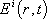. This incident field induces a current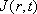on the surface S of the conductor that in turn radiates a scattered field. Enforcing the boundary condition on the total magnetic field or electric field on S gives rise to a time domain magnetic field integral equation (TDMFIE) or a time domain electric field integral equation (TDEFIE), respectively.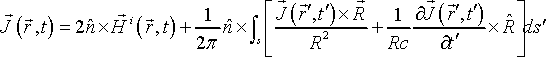(1)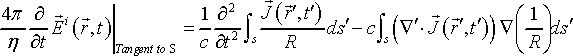(2)

Note that in the principal value, we essentially exclude the part where the source and observation points are the same (i.e. R=0). Since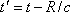, and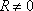, it is always true that. The main difficulty in extending the approach used to solve the frequency domain integral equations comes from the retarded time variable. However, the TDIEs can be solved numerically by means of a marching-on-in-time (MOT) procedure.

Like the method of moments in the frequency domain, the MoM-TD method discretizes the scatterers or targets into segments or patches. The time axis is then divided into equal increments or time steps. The triangular patches and vector basis functions proposed by Rao-Wilton-Glisson (RWG)  are commonly used to discretize the current in space and time by expanding the currentas a finite linear combination of products of spatial basis functions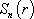and temporal basis functions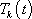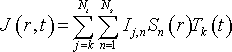(3)

where the temporal basis functions are generally versions of the same function shifted by a certain number of time steps,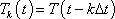with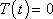,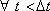.

To determine the expansion coefficients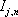, Galerkin testing functions are applied in space and point matching is applied at times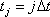,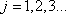, leading to a set of matrix equations that can be written as,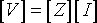(4)

The vector [V] contains the known incident field quantities and the terms of the Z-matrix are functions of the geometry. The unknown coefficients of the induced current are the terms of the [I] vector. These values are obtained by solving the system of equations iteratively. For example, Andriulli  proposed an explicit iterative scheme,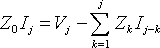(5)

where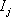is a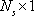vector,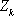is a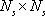matrix relating the currents on the body at time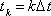. The current coefficient vectorcan be obtained once the current coefficient vectors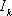,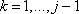are known.

The TDIEs have applications and limitations similar to their frequency domain counterparts. The EFIE is suitable for closed and open bodies, while the MFIE is only suitable for smooth, closed bodies. MoM-TD techniques are not very effective when applied to arbitrary configurations with complex geometries or inhomogeneous dielectrics. They also are not well-suited for analyzing the interior of conductive enclosures or thin plates with wire attachments on both sides. However, the time domain MoM is especially well suited for dealing with fast transient electromagnetic fields incident on or radiated from structures in free space.

## References

 S. M. Rao, T. K. Sarkar, and S. A. Dianat, "A novel technique to the solution of transient electromagnetic scattering from thin wires," IEEE Trans. Antennas Propagat., vol. 34, pp. 630-634, May 1986.

 A. R. Bretones, R. G. Martin, and A. Salinas, "DOTIG1, a time-domain numerical code for the study of the interaction of electromagnetic pulses with thin-wire structures," COMPEL, vol. 8, no. 1, pp. 39-61, 1989.

 S. M. Rao and D. R. Wilton, "Transient scattering by conducting surfaces of arbitrary shape," IEEE Trans. Antennas Propagat., vol. 39, no. 1, pp. 56-61, Jan. 1991.

 B. P. Rynne, "Time domain scattering from arbitrary surfaces using the electric field integral equation," J. Electromagn. Waves Appl., vol. 5, pp. 93-112, 1991.

 D. A. Vechinski and S. M. Rao, "A stable procedure to calculate the transient scattering by conducting surfaces of arbitrary shape," IEEE Trans. Antennas Propagat., vol. 40, pp. 661-665, June 1992.

 P. J. Davies, "Time-Marching numerical schemes for the electric field integral equation on a straight thin wire," Advances in Computational Mathematics, 2, 1994.

 G. Manara, A. Monorchio, R. Reggiannini, "A space-time discretization criterion for a stable time-marching solution of the electric field integral equation," IEEE Trans. Antennas Propagat., vol. 45, no. 3, March 1997.

 G. Cerri, R. de Leo, R. de Rentiis, V.M. Primiani, "ESD field penetration through slots into shielded enclosures: a time domain approach," IEEE Trans. on Electromag. Compat., vol. 39, no. 4, pp. 377-386, Nov. 1997.

 M. J. Bluck and S. P. Walker, "Time-domain BIE analysis of large three-dimensional electromagnetic scattering problems," IEEE Trans. Antennas Propagat., vol. 45, pp. 894-901, May 1997.

 S. M. Rao and T. K. Sarkar , "Time domain modeling of two dimensional conducting cylinders utilizing an implicit scheme," Microwave Opt. Technol. Lett., vol. 15, pp. 342-347, Aug. 1997.

 S. M. Rao and T. K. Sarkar, "Transient analysis of electromagnetic scattering from wire structures utilizing an implicit time domain integral equation technique," Microwave Opt. Technol. Lett., vol. 16, pp. 66-69, Jan. 1998.

 S. M. Rao and T. K. Sarkar, "An efficient method to evaluate the time-domain scattering from arbitrarily shaped conducting bodies," Microwave Opt. Technol. Lett., vol. 17, pp. 321-325, Apr. 1998.

 M. D. Pocock, M. J. Bluck, and S. P. Walker, "Electromagnetic scattering from 3-D curved dielectric bodies using time-domain integral equations," IEEE Trans. Antennas Propagat., vol. 46, pp. 1212-1219, Aug. 1998.

 S. M. Rao, D. A. Vechinski, and T. K. Sarkar, "Transient scattering by conducting cylinders: Implicit solution for transverse electric case," Microwave Opt. Technol. Lett., vol. 21, pp. 129-134, Apr. 1999.

 S. M. Rao and T. K. Sarkar, "Implicit solution of time domain integral equations for arbitrarily shaped dielectric bodies," Microwave Opt. Technol. Lett., vol. 21, pp. 201-205, May 1999.

 D. S. Weile, B. Shanker, E. Michielssen, "An accurate scheme for the numerical solution of the time domain electric field integral equation," Proc. of IEEE Int. Symp. on Antennas and Propagation, vol. 4, pp. 516-519, July 2001.

 P. Argus, H. Singer, "New basis functions for connections between wire and surface structures for time domain method of moments," Proc. IEEE Int. Symp. on Electromagnetic Compatibility, vol. 1, pp. 463 - 468, Aug. 2002.

 S. M. Rao and T. K. Sarkar, "Numerical solution of time domain integral equations for arbitrary shaped conductor/dielectric composite bodies," IEEE Trans. Antennas Propagat., vol. 50, no. 12, pp. 1831-1837, Dec. 2002.

 B. Shanker, A. A. Ergin, M. Lu, and E. Michielssen, "Fast analysis of transient electromagnetic scattering phenomena using the multilevel plane wave time domain algorithm," IEEE Trans. Antennas Propagat., vol. 51, no. 3, pp. 628-641, March 2003.

 R.A. Wildman, G. Pisharody, D.S. Weile, S. Balasubramaniam, and E. Michielssen, "An accurate scheme for the solution of the time-domain Integral equations of electromagnetics using higher order vector bases and band limited extrapolation," IEEE Trans. Antennas Propagat., vol. 52, no. 11, pp. 2973-2984, Nov. 2004.

 Y.S. Chung, T.K. Sarkar, B.H. Jung, M. S. Palma, Z. Ji, S. Jang, and K. Kim, "Solution of time domain electric field Integral equation using the Laguerre polynomials," IEEE Trans. Antennas Propagat., vol. 52, no. 9, pp. 2319-2328, Sep. 2004.

 D.S. Weile, G. Pisharody, N.W. Chen, B. Shanker, E. Michielssen, " A novel scheme for the solution of the time-domain integral equations of electromagnetics," IEEE Trans. Antennas Propagat., vol. 52, no. 1, pp. 283-295, Jan. 2004.

 Z. Ji, T.K. Sarkar, B.H. Jung, Y.S. Chung, M.S. Palma, and M. Yuan, "A stable solution of time domain electric field Integral equation for thin-wire antennas using the Laguerre polynomials," IEEE Trans. Antennas Propagat., vol. 52, no. 10, pp. 2641-2649, Oct. 2004.

 B. Jung, T. Sarkar, Y. Chung, M. Palma, Z. Ji, S. Jang, and K. Kim, "Transient electromagnetic scattering from dielectric objects using the electric field Integral equation with Laguerre polynomials as temporal basis functions," IEEE Trans. Antennas Propagat., vol. 52, no. 9, pp. 2329-2340, Sep. 2004.

 S. Wang, S. Chai, J. Mao, "A method to accelerate the MOT arithmetic in TD-MOM EFIE," Microwave Conference Proceedings, 2005. APMC 2005. Asia-Pacific Conference Proceedings, vol. 4, Dec. 2005.

 R.A. Lemdiasov, A.A. Obi, and R. Ludwig, "Time domain formulation of the method of moments for inhomogeneous conductive bodies at low frequencies," IEEE Trans. Antennas Propagat., vol. 54, no. 2, pp. 706-714, Feb. 2006.

 G. Guarnieri, S. Selleri, G. Pelosi, C. Dedeban and C. Pichot, "Innovative basis and weight functions for wire junctions in time domain moment method," IEE Proceedings -Microwaves, Antennas and Propagation, vol. 153, no. 1, pp. 61-66, Feb. 2006.

 F.P. Andriulli, H. Bagsi, F. Vipiana, G. Vecchi, and E. Michielssen, "A marching-on-in-time hierarchical scheme for the solution of the time domain electric field integral equation," IEEE Trans. Antennas Propagat., vol. 55, no. 12, pp. 3734-3738, Dec. 2007.

 L.J. Li, L. Li, C. Gao, X. Gu, and Z. Zhao, "Numerical Treatment for Time Domain Integral Equations of Thin Wire Structures in Half-Space Configuration," IEEE Trans. on Magnetics, vol. 44, no. 6, pp. 774-777, June 2008.

 G. Pisharody, and D.S. Weile, "An accurate solution to time domain integral equations for inhomogeneous dielectric bodies using higher-order volume bases," Proc. of IEEE Int. Symp.on Antennas and Propagation, pp. 2805-2808, July 2006.

 S. M. Rao, D. R. Wilton, and A. W. Glisson, "Electromagnetic scattering by surfaces of arbitrary shape," IEEE Trans. Antennas Propagat., vol. 30, pp. 409-418, May 1982.